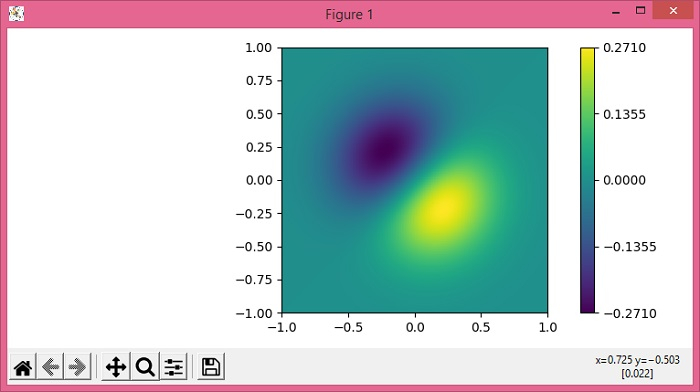# How to add Matplotlib Colorbar Ticks?

MatplotlibPythonData Visualization

To add ticks to the colorbar, we can take the following steps −

• Set the figure size and adjust the padding between and around the subplots.

• Create x, y and z data points using numpy.

• Use imshow() method to display the data as an image, i.e., on a 2D regular raster.

• Create ticks using numpy in the range of min and max of z.

• Create a colorbar for a ScalarMappable instance, *mappable*, with ticks=ticks.

• To display the figure, use show() method.

## Example

import numpy as np
import matplotlib.pyplot as plt

plt.rcParams["figure.figsize"] = [7.50, 3.50]
plt.rcParams["figure.autolayout"] = True

x, y = np.mgrid[-1:1:100j, -1:1:100j]
z = (x + y) * np.exp(-5.0 * (x ** 2 + y ** 2))

plt.imshow(z, extent=[-1, 1, -1, 1])

ticks = np.linspace(z.min(), z.max(), 5, endpoint=True)

cb = plt.colorbar(ticks=ticks)

plt.show()

## Output